# CPU#

## ALU#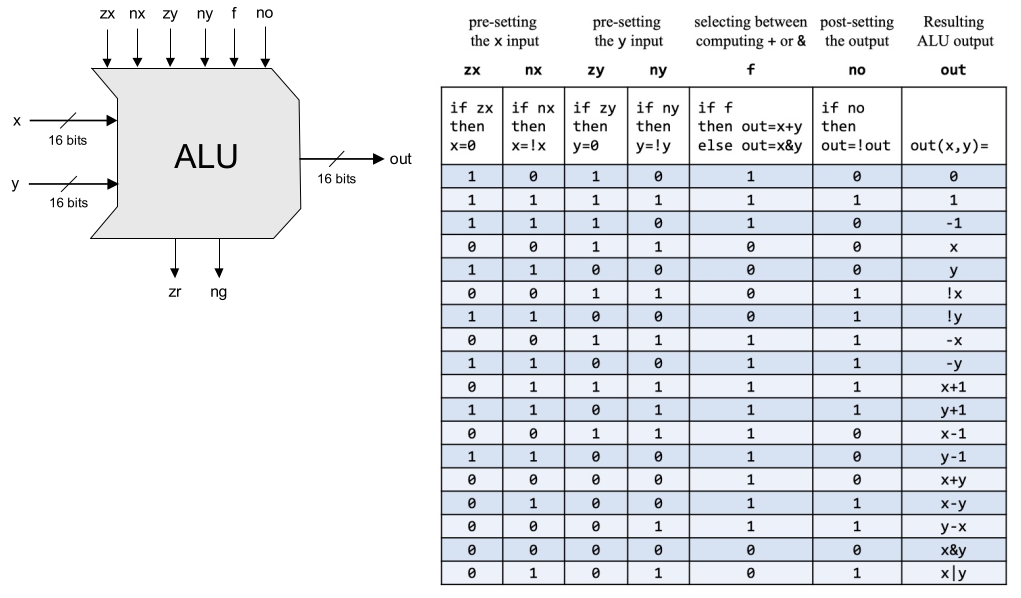• zx：選擇 input 1 或 0 作為 x
• nx：not x
• zy：選擇 input2 或 0 作為 y
• ny：not y
• f：out = x+y 或 x&y
• no：not out

``````module hack_alu (
input i_zx,
input i_nx,
input i_zy,
input i_ny,
input i_f,
input i_no,
input [15:0] inx,
input [15:0] iny,
output logic o_zero,
output logic o_negative,
output logic [15:0] out
);

logic [15:0] zerox;
logic [15:0] notx;
logic [15:0] zeroy;
logic [15:0] noty;
logic [15:0] fout;

assign zerox = i_zx ? 0 : inx;
assign notx = i_nx ? ~zerox : zerox;
assign zeroy = i_zy ? 0 : iny;
assign noty = i_ny ? ~zeroy : zeroy;
assign fout = i_f ? notx + noty : notx & noty;
assign out = i_no ? ~fout : fout;

assign o_zero = out == 0;
assign o_negative = out == 1;

endmodule
``````

## Register 和 PC#

register 跟 pc 是 sequential 的元件，會在 clk 來的時候把 input 值存入 flip-flop：

``````module hack_pc (
input clk,
input rst,
input inc,
input [15:0] in,
output logic [15:0] out
);

always_ff @(posedge clk or negedge rst) begin
if (!rst) begin
out <= 0;
end
out <= in;
end
else if (inc) begin
out <= out + 1;
end
end
endmodule
``````

``````module register (
input clk,
input [15:0] in,
output logic [15:0] out
);

always_ff @(posedge clk) begin
out <= in;
end
end

endmodule
``````

## CPU#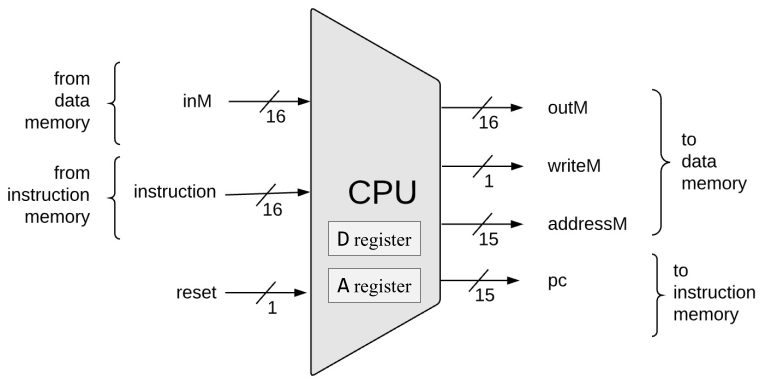Nand2Tetris CPU 的介面如下：

• rst：重設 CPU
• instruction：來自 ROM 的 instruction
• inM：從 RAM 讀取指定記憶體位址的內容
• pc：下一個從 ROM 中取 instruction 的位址

``````module hack_cpu (
input clk,
input rst,
input [15:0] instruction,
input [15:0] i_memory,
output logic o_we,
output logic [15:0] o_out,
output logic [15:0] o_pc
);
``````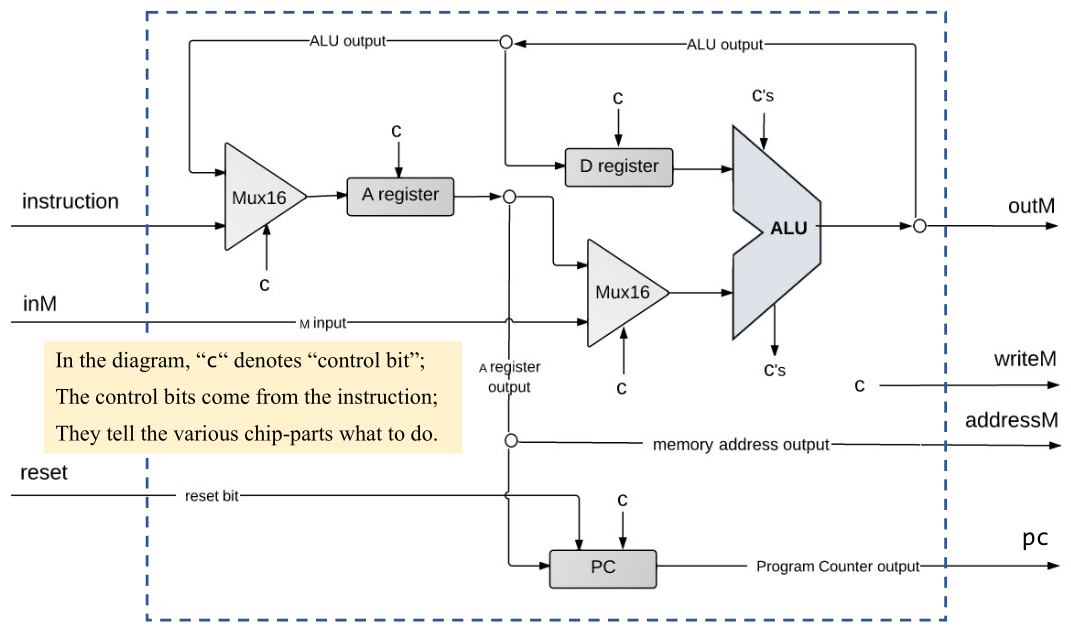Hack CPU 的架構圖如上，裡面有兩個 register，D (Data) Register 跟 A (Address) Register； 顧名思義，A register 的值表示的就是現在要存取 Memory 的位址；D Register 則是運算用的資料。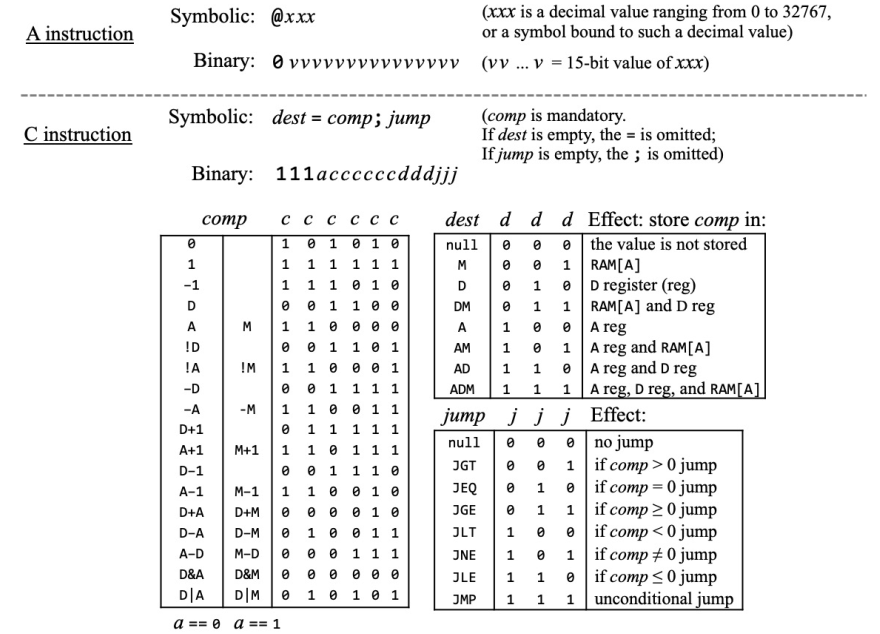Hack CPU 只有兩種指令：

1. A instruction，開頭為 0 表示，把後面的 15 bits 的數值存入 A register。
2. C instruction，開頭為 1，後面幾個 bits 分別代表：
• a：選擇 ALU 的輸入是 A register 或 memory input。
• c：決定 ALU 要進行的運算。
• d：Destnation，運算結果要是否要寫入 A register/D register/RAM 裡面。
• j：Jump，依運算結果是否進行跳轉。

``````logic [15:0] inx;
logic [15:0] iny;
logic [15:0] a_out;
logic [15:0] alu_out;
logic [15:0] next_pc;
logic f_zero;
logic f_negative;
logic jump;

logic is_A_inst, is_C_inst;
assign is_A_inst = (instruction == 0);
assign is_C_inst = !is_A_inst;

assign addr_instruction = is_A_inst ? instruction : alu_out;

always_comb begin
if (is_C_inst) begin
case ({instruction, instruction, instruction})
3'b000: jump = 0;
3'b001: jump = !(f_negative || f_zero);
3'b010: jump = f_zero;
3'b011: jump = !f_negative;
3'b100: jump = f_negative;
3'b101: jump = !f_zero;
3'b110: jump = f_negative || f_zero;
3'b111: jump = 1;
endcase
end
else begin
jump = 0;
end
end
``````

``````hack_pc pc (
.clk(clk), .rst(rst),
.in(a_out + 1), .out(next_pc)
);

register register_d (
.clk(clk),
.in(alu_out),
.out(inx)
);

register register_a (
.clk(clk),
.out(a_out)
);

hack_alu alu (
.i_zx(instruction),
.i_nx(instruction),
.i_zy(instruction),
.i_ny(instruction),
.i_f(instruction),
.i_no(instruction),
.inx(inx),
.iny(iny),
.o_zero(f_zero),
.o_negative(f_negative),
.out(alu_out)
);

assign o_out = alu_out;
assign o_we = !is_A_inst && instruction;
assign o_pc = jump ? a_out : next_pc;
``````

# Memory#

Memory 應該是本次最大的麻煩，nand2tetris 在設計上，每個位址有 16 bits 的資料：

1. ROM 定址 32 K
2. RAM 定址 16 K
3. display memory 定址 8 K
4. 1 byte 的鍵盤輸入，這個我們先不管它

1. ROM 定址 16K
2. RAM 定址 16K
3. display memory 定址 8K + 8K 總共 48 K。

## ROM#

``````module rom (
input clk,
output logic [WIDTH-1:0] data
);

parameter WIDTH=16;
parameter DEPTH=14;
parameter SIZE=(1<<DEPTH);
parameter FILE="rom.hack";

logic [WIDTH-1:0] ram [0:SIZE-1];

`ifdef verilator
initial begin
end
`else
initial begin
if (FILE) begin
end
end
`endif

always_ff @(posedge clk) begin
end

endmodule
``````

Instruction, ROM output next PC PC output
n+1 k+1 k
k k+2 k+1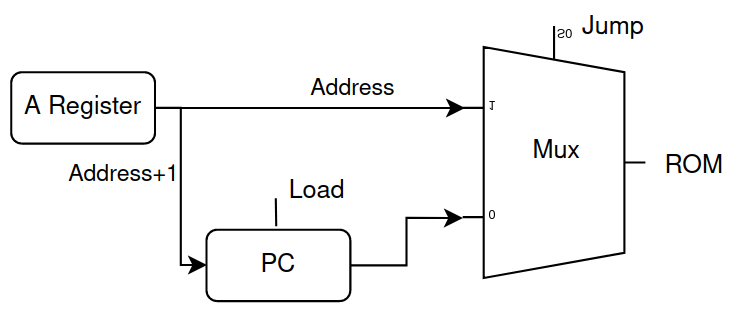## RAM#

RAM 就沒什麼特別的了，宣告 16K 的 ram 和 8K 的 screen，CPU 的存取會分別導向這兩塊 memory；GPU 則只存取 screen。

``````module ram (
input clk,

// CPU rw side
input we,
output logic [WIDTH-1:0] data_rd,
input [WIDTH-1:0] data_wr,

output logic [WIDTH-1:0] gpu_data
);

localparam WIDTH = 16;
localparam DEPTH = 16;
localparam RAM_SIZE = (1<<14);
localparam SCREEN_SIZE = (1<<13);

// 16K cpu ram
logic [WIDTH-1:0] ram [0:RAM_SIZE-1];
// 8K screen ram
logic [WIDTH-1:0] screen [0:SCREEN_SIZE-1];

logic [WIDTH-1:0] ram_data;
logic [WIDTH-1:0] screen_data;

// CPU side
always_comb begin
end

always_ff @(posedge clk) begin
if (we && addr[15:14] == 2'b00) begin
end
end

always_comb begin
end

always_ff @(posedge clk) begin
if (we && addr[15:14] == 2'b01) begin
end
end

always_comb begin
data_rd = ram_data;
end
else if (addr[15:14] == 2'b01) begin
data_rd = screen_data;
end
else begin
data_rd = 0;
end
end

// GPU side
always_ff @(posedge clk) begin
end

endmodule
``````

# top module#

``````logic [15:0] rom_addr;
logic [15:0] instruction;
logic [15:0] mem_out;
logic mem_we;

rom #(
.WIDTH(16),
.DEPTH(14),
.SIZE(1<<14),
.FILE("rom.hack")
) rom_i (
.clk(clk),
);

hack_cpu cpu (
.clk(clk), .rst(rst),
.instruction(instruction), .i_memory(mem_out),
.o_we(mem_we), .o_out(cpu_out),
);

ram ram_i (
.clk(clk),
.we(mem_we),
);
``````

Nand2Tetris 一個 pixel 就是一個 bit，螢幕尺寸為 512x256，總共有 131072 pixel 也就是 RAM 定址 8K x 每個位址 16 bits 的內容。

``````assign gpu_addr = (counterY << 5) + (counterX >> 4);

always_comb begin
if (counterX >= 512 || counterY >= 256) begin
pixel = 24'd0;
end
else begin
pixel = (gpu_data[counterX & 4'hf]) ? 24'hffffff : 0;
end
end
``````

# 除錯#

1. 第一個是沒考慮到 leading/trailing space 造成 jump 字串的判斷錯誤，asm 寫 jump 結果沒有 jump。
2. 另一個是沒處理 A+D 跟 D+A、D+M 跟 M+D 的差別，一樣吐了錯誤的 command 出來。

# 執行#

## Sys.jack#

``````class Sys {
/** Performs all the initializations required by the OS. */
function void init() {
do Screen.init();
do Main.main();
do halt();
}

/** Halts execution. */
function void halt() {
while (true) {
}
}

function void oneMilliSecond() {
var int i;
let i = 0;
while (i < 275) {
let i = i + 1;
}
return;
}

function void wait(int duration) {
var int i;
let i = 0;
while (i < duration) {
do oneMilliSecond();
let i = i + 1;
}
return;
}
}
``````

Sys 提供了 oneMilliSecond 函式和 wait 函式，oneMilliSecond 的 275 是實驗出來的，理論上可以去查找 assembly， 看看這個 while loop 會執行多少個指令，然後去算執行 25000 個指令要執行多少次迴圈，不過我懶…畢竟我自己寫的編譯器編出來的 code 沒人看得懂…。

## Screen.jack#

``````class Screen {
static array SCREEN;
static int SCRSIZ;
static boolean COLOR;

/** Initializes the Screen. */
function void init() {
let SCREEN = 16384;
let SCRSIZ = 8192;
let COLOR = true;
return;
}

function void fillScreen(bool on) {
var int i;
let i = 0;
while(i < SCRSIZ) {
let SCREEN[i] = on;
let i = i + 1;
}
return;
}
}
``````

Screen 本來會提供更多必要的繪圖函式，刪掉只留下 fillScreen 把整個全螢幕變黑或變白，作為測試之用。

## Main.jack#

``````class Main {
function void main() {
while(true) {
do Screen.fillScreen(true);
do Sys.wait(1000);
do Screen.fillScreen(false);
do Sys.wait(1000);
}
return;
}
}
``````

Main 函式就是不斷的變白變黑。

# nand2tetris 範例#

nand2tetris 內部有三個範例程式

1. Screen
2. Output
3. String

phoning：沒有看波形解不掉的問題，如果…沒有如果！沒有就是沒有！

``````// CPU side
always_comb begin
end

// 第一個問題就是 screen_data 這裡寫錯，寫成 always_ff 資料就慢了一個 cycle
always_comb begin
end

// GPU side
always_ff @(posedge clk) begin
end
``````

``````logic [3:0] index;

always_comb begin
if (counterX >= 512 || counterY >= 256) begin
o_pixel = 24'd0;
end
else begin
o_pixel = (i_data[index]) ? 24'hffffff : 0;
end
end
``````# 結語#

1. 讓 yosys 支援 dual port memory，這樣 display 就不用用到兩倍只要 8K 就夠，但以 issue 卡關的程度來看，應該不是短期能辦到的事。
2. 設法接通 DRAM，這樣 block memory 56K 全部拿來當 ROM，但這個也是有點難度。
3. Xilinx 課金下去就對了，課金治百病